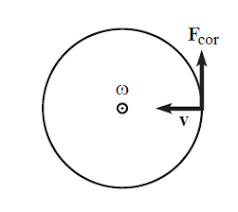## How to Calculate and Solve for Number of Revolutions per Minute and Angular Velocity of Motion of Circular Path | The Calculator EncyclopediaThe image above represent angular velocity.

To compute the angular velocity, one essential parameter is needed and its parameter is Number of Revolutions per Minute (N).

The formula for calculating angular velocity:

ω = 2πN / 60

Where;
ω = Angular velocity
N = Number of revolutions per minute

Let’s solve an example;
Find the Angular Velocity with a number of revolutions per minute as 60.

This implies that;
N = Number of revolutions per minute = 60

ω = 2πN / 60
ω = 2 x π x 24 / 60
ω = 150.816/ 60
ω = 2.5136

Therefore, the angular velocity is 2.5136 rad/s.

Calculating the Number of Revolutions per Minute when Angular Velocity is Given.

N = ω60 /

Where;
N = Number of revolutions per minute
ω = Angular velocity

Let’s solve an example;
With an angular velocity of 40. Find the number of revolutions per minute?

This implies that;
ω = Angular velocity = 40

N = ω60 /
N = 40 x 60 / 6.284
N = 2400 / 6.284
N = 381.9

Therefore, the number of revolutions per minute is 381.9 min.